I’ve never worked in the field of computer vision and has no idea how the magic could work when an autonomous car is configured to tell apart a stop sign from a pedestrian in a red hat. To motivate myself to look into the maths behind object recognition and detection algorithms, I’m writing a few posts on this topic “Object Detection for Dummies”. This post, part 1, starts with super rudimentary concepts in image processing and a few methods for image segmentation. Nothing related to deep neural networks yet. Deep learning models for object detection and recognition will be discussed in Part 2 and Part 3.

Disclaimer: When I started, I was using “object recognition” and “object detection” interchangeably. I don’t think they are the same: the former is more about telling whether an object exists in an image while the latter needs to spot where the object is. However, they are highly related and many object recognition algorithms lay the foundation for detection.

Links to all the posts in the series: [Part 1] [Part 2] [Part 3] [Part 4].

First of all, I would like to make sure we can distinguish the following terms. They are very similar, closely related, but not exactly the same.

Value type Scalar Scalar Vector
Definition The rate of change of a function $f(x,y,z,…)$ at a point $(x_0,y_0,z_0,…)$, which is the slope of the tangent line at the point. The instantaneous rate of change of $f(x,y,z, …)$ in the direction of an unit vector $\vec{u}$. It points in the direction of the greatest rate of increase of the function, containing all the partial derivative information of a multivariable function.

In the image processing, we want to know the direction of colors changing from one extreme to the other (i.e. black to white on a grayscale image). Therefore, we want to measure “gradient” on pixels of colors. The gradient on an image is discrete because each pixel is independent and cannot be further split.

The image gradient vector is defined as a metric for every individual pixel, containing the pixel color changes in both x-axis and y-axis. The definition is aligned with the gradient of a continuous multi-variable function, which is a vector of partial derivatives of all the variables. Suppose f(x, y) records the color of the pixel at location (x, y), the gradient vector of the pixel (x, y) is defined as follows:

\begin{align*} \nabla f(x, y) = \begin{bmatrix} g_x \\ g_y \end{bmatrix} = \begin{bmatrix} \frac{\partial f}{\partial x} \\[6pt] \frac{\partial f}{\partial y} \end{bmatrix} = \begin{bmatrix} f(x+1, y) - f(x-1, y)\\ f(x, y+1) - f(x, y-1) \end{bmatrix} \end{align*}

The $\frac{\partial f}{\partial x}$ term is the partial derivative on the x-direction, which is computed as the color difference between the adjacent pixels on the left and right of the target, f(x+1, y) - f(x-1, y). Similarly, the $\frac{\partial f}{\partial y}$ term is the partial derivative on the y-direction, measured as f(x, y+1) - f(x, y-1), the color difference between the adjacent pixels above and below the target.

There are two important attributes of an image gradient:

• Magnitude is the L2-norm of the vector, $g = \sqrt{ g_x^2 + g_y^2 }$.
• Direction is the arctangent of the ratio between the partial derivatives on two directions, $\theta = \arctan{(g_y / g_x)}$.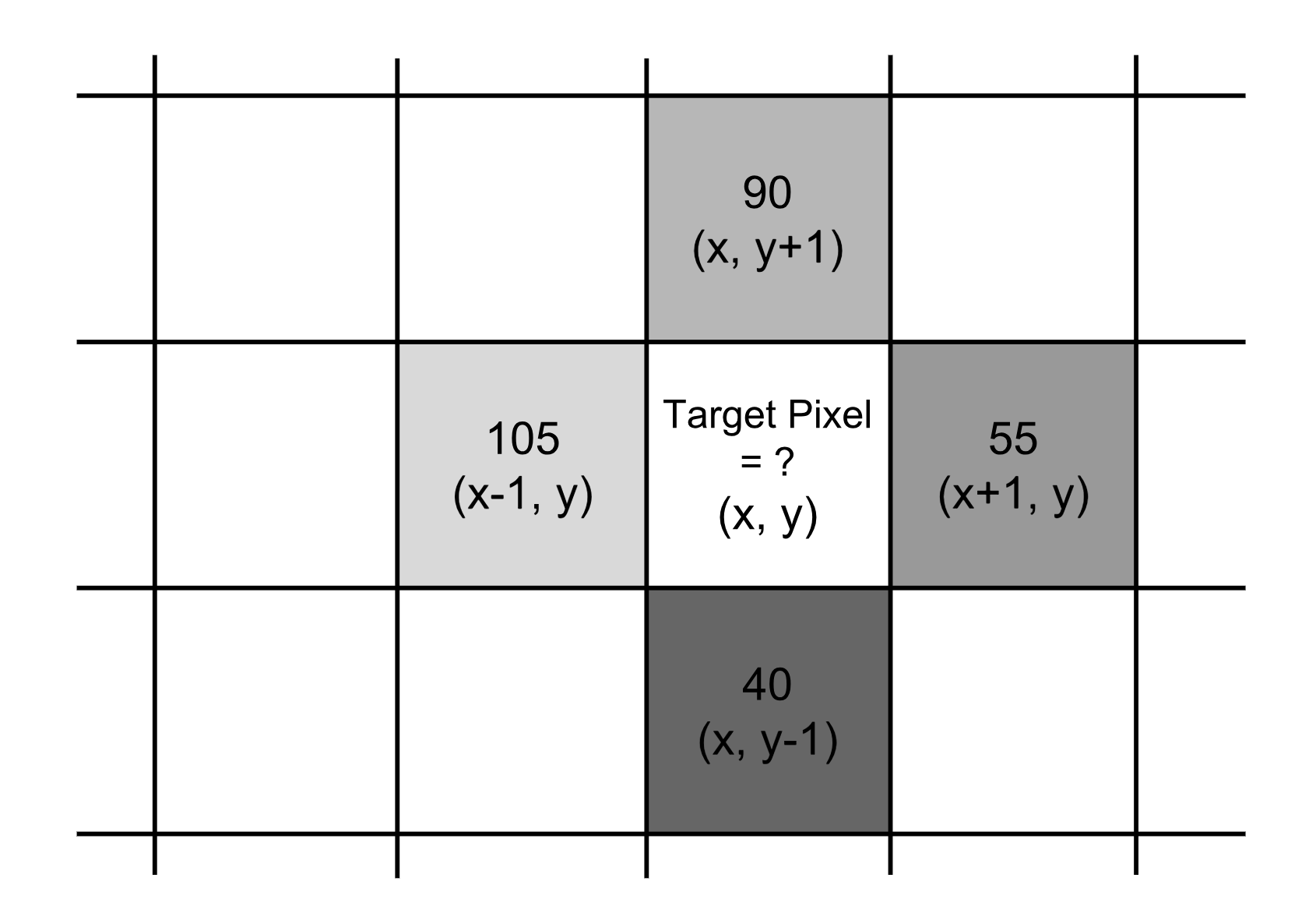The gradient vector of the example in Fig. 1. is:

\begin{align*} \nabla f = \begin{bmatrix} f(x+1, y) - f(x-1, y)\\ f(x, y+1) - f(x, y-1) \end{bmatrix} = \begin{bmatrix} 55-105\\ 90-40 \end{bmatrix} = \begin{bmatrix} -50\\ 50 \end{bmatrix} \end{align*}

Thus,

• the magnitude is $\sqrt{50^2 + (-50)^2} = 70.7107$, and
• the direction is $\arctan{(-50/50)} = -45^{\circ}$.

Repeating the gradient computation process for every pixel iteratively is too slow. Instead, it can be well translated into applying a convolution operator on the entire image matrix, labeled as $\mathbf{A}$ using one of the specially designed convolutional kernels.

Let’s start with the x-direction of the example in Fig 1. using the kernel $[-1,0,1]$ sliding over the x-axis; $\ast$ is the convolution operator:

\begin{align*} \mathbf{G}_x &= [-1, 0, 1] \ast [105, 255, 55] = -105 + 0 + 55 = -50 \end{align*}

Similarly, on the y-direction, we adopt the kernel $[+1, 0, -1]^\top$:

\begin{align*} \mathbf{G}_y &= [+1, 0, -1]^\top \ast \begin{bmatrix} 90\\ 255\\ 40 \end{bmatrix} = 90 + 0 - 40 = 50 \end{align*}

Try this in python:

import numpy as np
import scipy.signal as sig
data = np.array([[0, 105, 0], [40, 255, 90], [0, 55, 0]])
G_x = sig.convolve2d(data, np.array([[-1, 0, 1]]), mode='valid')
G_y = sig.convolve2d(data, np.array([[-1], , ]), mode='valid')


These two functions return array([, [-50], ]) and array([[0, 50, 0]]) respectively. (Note that in the numpy array representation, 40 is shown in front of 90, so -1 is listed before 1 in the kernel correspondingly.)

## Common Image Processing Kernels#

Prewitt operator: Rather than only relying on four directly adjacent neighbors, the Prewitt operator utilizes eight surrounding pixels for smoother results.

$$\mathbf{G}_x = \begin{bmatrix} -1 & 0 & +1 \\ -1 & 0 & +1 \\ -1 & 0 & +1 \end{bmatrix} \ast \mathbf{A} \text{ and } \mathbf{G}_y = \begin{bmatrix} +1 & +1 & +1 \\ 0 & 0 & 0 \\ -1 & -1 & -1 \end{bmatrix} \ast \mathbf{A}$$

Sobel operator: To emphasize the impact of directly adjacent pixels more, they get assigned with higher weights.

$$\mathbf{G}_x = \begin{bmatrix} -1 & 0 & +1 \\ -2 & 0 & +2 \\ -1 & 0 & +1 \end{bmatrix} \ast \mathbf{A} \text{ and } \mathbf{G}_y = \begin{bmatrix} +1 & +2 & +1 \\ 0 & 0 & 0 \\ -1 & -2 & -1 \end{bmatrix} \ast \mathbf{A}$$

Different kernels are created for different goals, such as edge detection, blurring, sharpening and many more. Check this wiki page for more examples and references.

## Example: Manu in 2004#

Let’s run a simple experiment on the photo of Manu Ginobili in 2004 [[Download Image]({{ ‘/assets/data/manu-2004.jpg’ | relative_url }}){:target="_blank"}] when he still had a lot of hair. For simplicity, the photo is converted to grayscale first. For colored images, we just need to repeat the same process in each color channel respectively.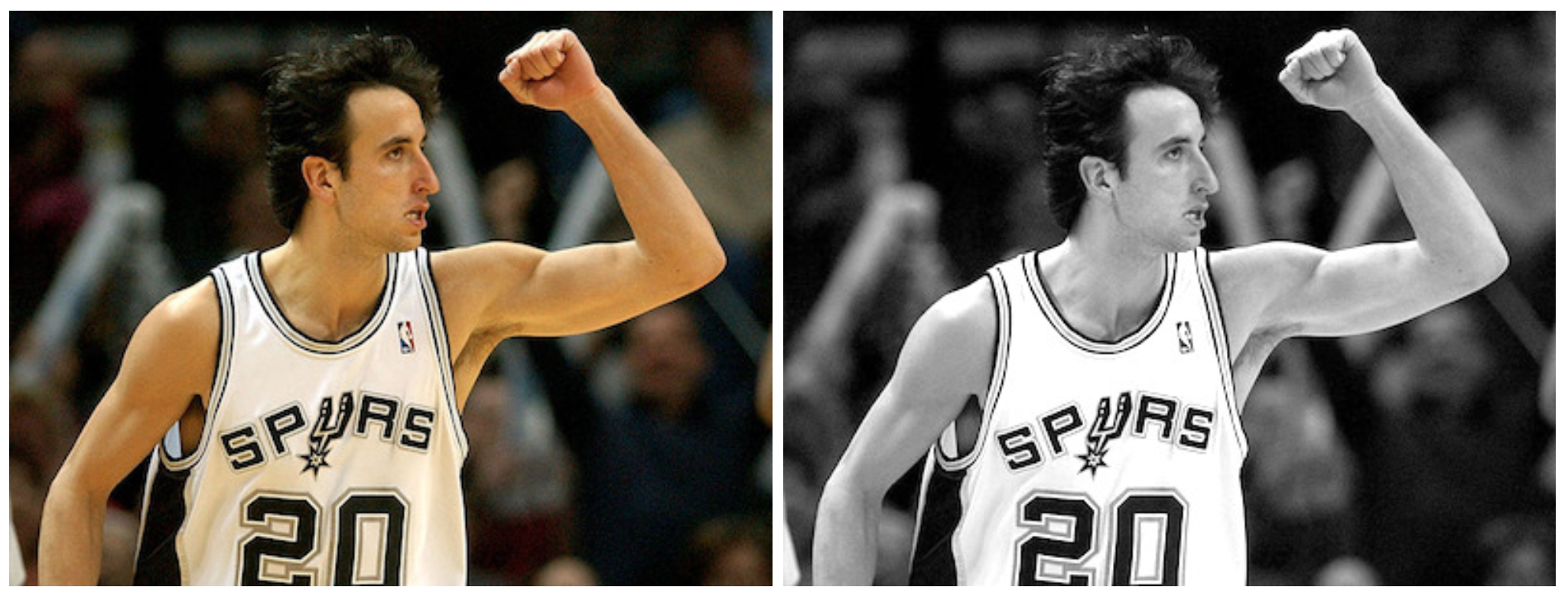import numpy as np
import scipy
import scipy.signal as sig
# With mode="L", we force the image to be parsed in the grayscale, so it is
# actually unnecessary to convert the photo color beforehand.

# Define the Sobel operator kernels.
kernel_x = np.array([[-1, 0, 1],[-2, 0, 2],[-1, 0, 1]])
kernel_y = np.array([[1, 2, 1], [0, 0, 0], [-1, -2, -1]])

G_x = sig.convolve2d(img, kernel_x, mode='same')
G_y = sig.convolve2d(img, kernel_y, mode='same')

# Plot them!
fig = plt.figure()

# Actually plt.imshow() can handle the value scale well even if I don't do
# the transformation (G_x + 255) / 2.
ax1.imshow((G_x + 255) / 2, cmap='gray'); ax1.set_xlabel("Gx")
ax2.imshow((G_y + 255) / 2, cmap='gray'); ax2.set_xlabel("Gy")
plt.show()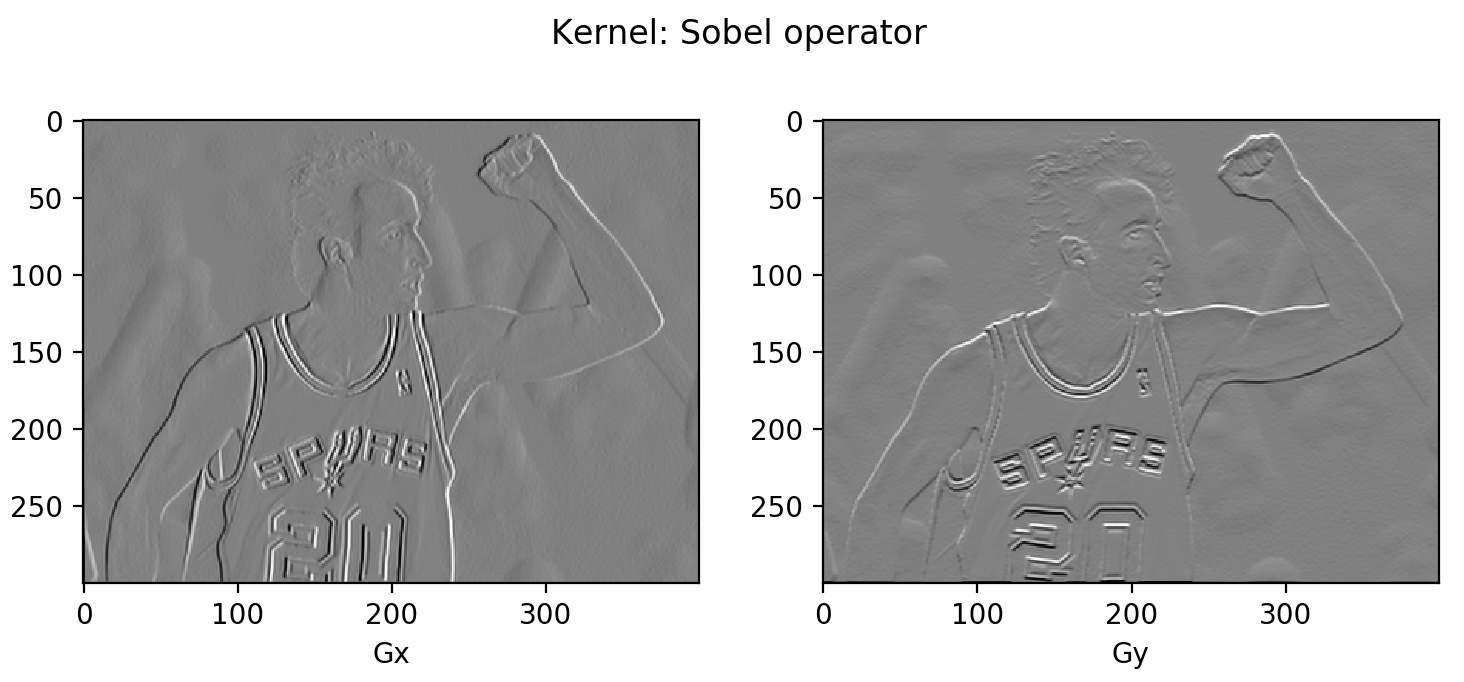You might notice that most area is in gray. Because the difference between two pixel is between -255 and 255 and we need to convert them back to [0, 255] for the display purpose. A simple linear transformation ($\mathbf{G}$ + 255)/2 would interpret all the zeros (i.e., constant colored background shows no change in gradient) as 125 (shown as gray).

# Histogram of Oriented Gradients (HOG)#

The Histogram of Oriented Gradients (HOG) is an efficient way to extract features out of the pixel colors for building an object recognition classifier. With the knowledge of image gradient vectors, it is not hard to understand how HOG works. Let’s start!

## How HOG works#

1. Preprocess the image, including resizing and color normalization.

2. Compute the gradient vector of every pixel, as well as its magnitude and direction.

3. Divide the image into many 8x8 pixel cells. In each cell, the magnitude values of these 64 cells are binned and cumulatively added into 9 buckets of unsigned direction (no sign, so 0-180 degree rather than 0-360 degree; this is a practical choice based on empirical experiments).

For better robustness, if the direction of the gradient vector of a pixel lays between two buckets, its magnitude does not all go into the closer one but proportionally split between two. For example, if a pixel’s gradient vector has magnitude 8 and degree 15, it is between two buckets for degree 0 and 20 and we would assign 2 to bucket 0 and 6 to bucket 20.

This interesting configuration makes the histogram much more stable when small distortion is applied to the image.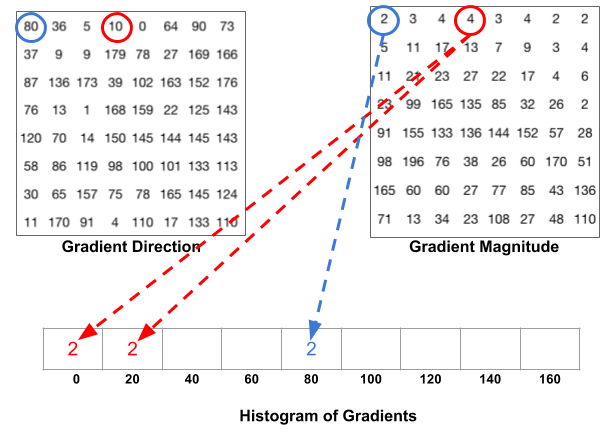1. Then we slide a 2x2 cells (thus 16x16 pixels) block across the image. In each block region, 4 histograms of 4 cells are concatenated into one-dimensional vector of 36 values and then normalized to have an unit weight. The final HOG feature vector is the concatenation of all the block vectors. It can be fed into a classifier like SVM for learning object recognition tasks.

## Example: Manu in 2004#

Let’s reuse the same example image in the previous section. Remember that we have computed $\mathbf{G}_x$ and $\mathbf{G}_y$ for the whole image.

N_BUCKETS = 9
CELL_SIZE = 8  # Each cell is 8x8 pixels
BLOCK_SIZE = 2  # Each block is 2x2 cells

def assign_bucket_vals(m, d, bucket_vals):
left_bin = int(d / 20.)
# Handle the case when the direction is between [160, 180)
right_bin = (int(d / 20.) + 1) % N_BUCKETS
assert 0 <= left_bin < right_bin < N_BUCKETS

left_val= m * (right_bin * 20 - d) / 20
right_val = m * (d - left_bin * 20) / 20
bucket_vals[left_bin] += left_val
bucket_vals[right_bin] += right_val

def get_magnitude_hist_cell(loc_x, loc_y):
# (loc_x, loc_y) defines the top left corner of the target cell.
cell_x = G_x[loc_x:loc_x + CELL_SIZE, loc_y:loc_y + CELL_SIZE]
cell_y = G_y[loc_x:loc_x + CELL_SIZE, loc_y:loc_y + CELL_SIZE]
magnitudes = np.sqrt(cell_x * cell_x + cell_y * cell_y)
directions = np.abs(np.arctan(cell_y / cell_x) * 180 / np.pi)

buckets = np.linspace(0, 180, N_BUCKETS + 1)
bucket_vals = np.zeros(N_BUCKETS)
map(
lambda (m, d): assign_bucket_vals(m, d, bucket_vals),
zip(magnitudes.flatten(), directions.flatten())
)
return bucket_vals

def get_magnitude_hist_block(loc_x, loc_y):
# (loc_x, loc_y) defines the top left corner of the target block.
return reduce(
lambda arr1, arr2: np.concatenate((arr1, arr2)),
[get_magnitude_hist_cell(x, y) for x, y in zip(
[loc_x, loc_x + CELL_SIZE, loc_x, loc_x + CELL_SIZE],
[loc_y, loc_y, loc_y + CELL_SIZE, loc_y + CELL_SIZE],
)]
)


The following code simply calls the functions to construct a histogram and plot it.

# Random location [200, 200] as an example.
loc_x = loc_y = 200

ydata = get_magnitude_hist_block(loc_x, loc_y)
ydata = ydata / np.linalg.norm(ydata)

xdata = range(len(ydata))
bucket_names = np.tile(np.arange(N_BUCKETS), BLOCK_SIZE * BLOCK_SIZE)

assert len(ydata) == N_BUCKETS * (BLOCK_SIZE * BLOCK_SIZE)
assert len(bucket_names) == len(ydata)

plt.figure(figsize=(10, 3))
plt.bar(xdata, ydata, align='center', alpha=0.8, width=0.9)
plt.xticks(xdata, bucket_names * 20, rotation=90)
plt.xlabel('Direction buckets')
plt.ylabel('Magnitude')
plt.grid(ls='--', color='k', alpha=0.1)
plt.title("HOG of block at [%d, %d]" % (loc_x, loc_y))
plt.tight_layout()


In the code above, I use the block with top left corner located at [200, 200] as an example and here is the final normalized histogram of this block. You can play with the code to change the block location to be identified by a sliding window.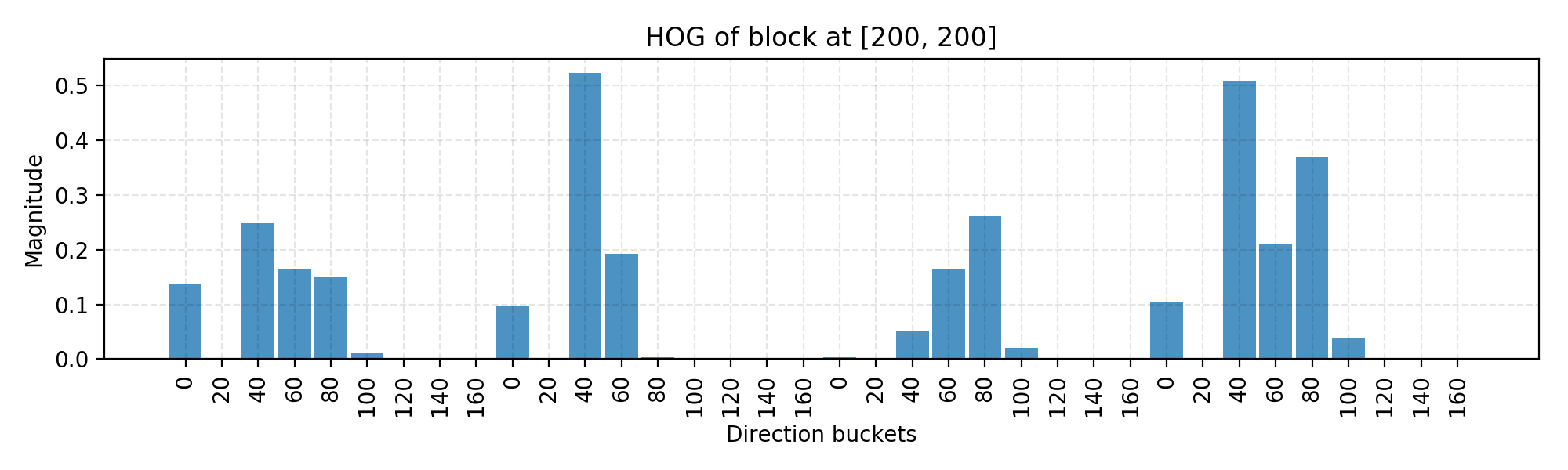The code is mostly for demonstrating the computation process. There are many off-the-shelf libraries with HOG algorithm implemented, such as OpenCV, SimpleCV and scikit-image.

# Image Segmentation (Felzenszwalb’s Algorithm)#

When there exist multiple objects in one image (true for almost every real-world photos), we need to identify a region that potentially contains a target object so that the classification can be executed more efficiently.

Felzenszwalb and Huttenlocher (2004) proposed an algorithm for segmenting an image into similar regions using a graph-based approach. It is also the initialization method for Selective Search (a popular region proposal algorithm) that we are gonna discuss later.

Say, we use a undirected graph $G=(V, E)$ to represent an input image. One vertex $v_i \in V$ represents one pixel. One edge $e = (v_i, v_j) \in E$ connects two vertices $v_i$ and $v_j$. Its associated weight $w(v_i, v_j)$ measures the dissimilarity between $v_i$ and $v_j$. The dissimilarity can be quantified in dimensions like color, location, intensity, etc. The higher the weight, the less similar two pixels are. A segmentation solution $S$ is a partition of $V$ into multiple connected components, $\{C\}$. Intuitively similar pixels should belong to the same components while dissimilar ones are assigned to different components.

## Graph Construction#

There are two approaches to constructing a graph out of an image.

• Grid Graph: Each pixel is only connected with surrounding neighbours (8 other cells in total). The edge weight is the absolute difference between the intensity values of the pixels.
• Nearest Neighbor Graph: Each pixel is a point in the feature space (x, y, r, g, b), in which (x, y) is the pixel location and (r, g, b) is the color values in RGB. The weight is the Euclidean distance between two pixels’ feature vectors.

## Key Concepts#

Before we lay down the criteria for a good graph partition (aka image segmentation), let us define a couple of key concepts:

• Internal difference: $Int(C) = \max_{e\in MST(C, E)} w(e)$, where $MST$ is the minimum spanning tree of the components. A component $C$ can still remain connected even when we have removed all the edges with weights < $Int(C)$.
• Difference between two components: $Dif(C_1, C_2) = \min_{v_i \in C_1, v_j \in C_2, (v_i, v_j) \in E} w(v_i, v_j)$. $Dif(C_1, C_2) = \infty$ if there is no edge in-between.
• Minimum internal difference: $MInt(C_1, C_2) = min(Int(C_1) + \tau(C_1), Int(C_2) + \tau(C_2))$, where $\tau(C) = k / \vert C \vert$ helps make sure we have a meaningful threshold for the difference between components. With a higher $k$, it is more likely to result in larger components.

The quality of a segmentation is assessed by a pairwise region comparison predicate defined for given two regions $C_1$ and $C_2$:

$$D(C_1, C_2) = \begin{cases} \text{True} & \text{ if } Dif(C_1, C_2) > MInt(C_1, C_2) \\ \text{False} & \text{ otherwise} \end{cases}$$

Only when the predicate holds True, we consider them as two independent components; otherwise the segmentation is too fine and they probably should be merged.

## How Image Segmentation Works#

The algorithm follows a bottom-up procedure. Given $G=(V, E)$ and $|V|=n, |E|=m$:

1. Edges are sorted by weight in ascending order, labeled as $e_1, e_2, \dots, e_m$.
2. Initially, each pixel stays in its own component, so we start with $n$ components.
3. Repeat for $k=1, \dots, m$:
• The segmentation snapshot at the step $k$ is denoted as $S^k$.
• We take the k-th edge in the order, $e_k = (v_i, v_j)$.
• If $v_i$ and $v_j$ belong to the same component, do nothing and thus $S^k = S^{k-1}$.
• If $v_i$ and $v_j$ belong to two different components $C_i^{k-1}$ and $C_j^{k-1}$ as in the segmentation $S^{k-1}$, we want to merge them into one if $w(v_i, v_j) \leq MInt(C_i^{k-1}, C_j^{k-1})$; otherwise do nothing.

If you are interested in the proof of the segmentation properties and why it always exists, please refer to the paper.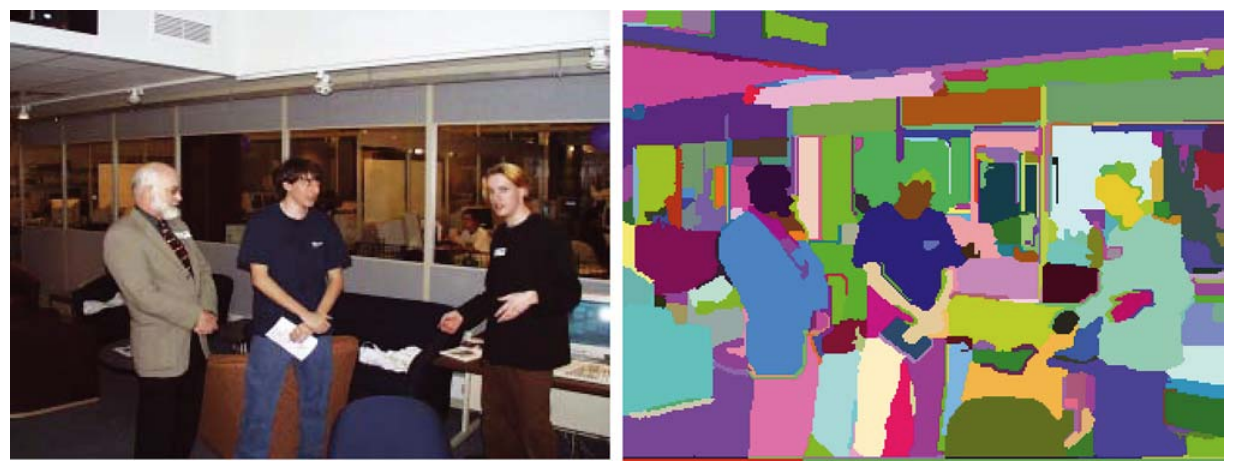## Example: Manu in 2013#

This time I would use the photo of old Manu Ginobili in 2013 [[Image]({{ ‘/assets/data/manu-2013.jpg’ | relative_url }})] as the example image when his bald spot has grown up strong. Still for simplicity, we use the picture in grayscale.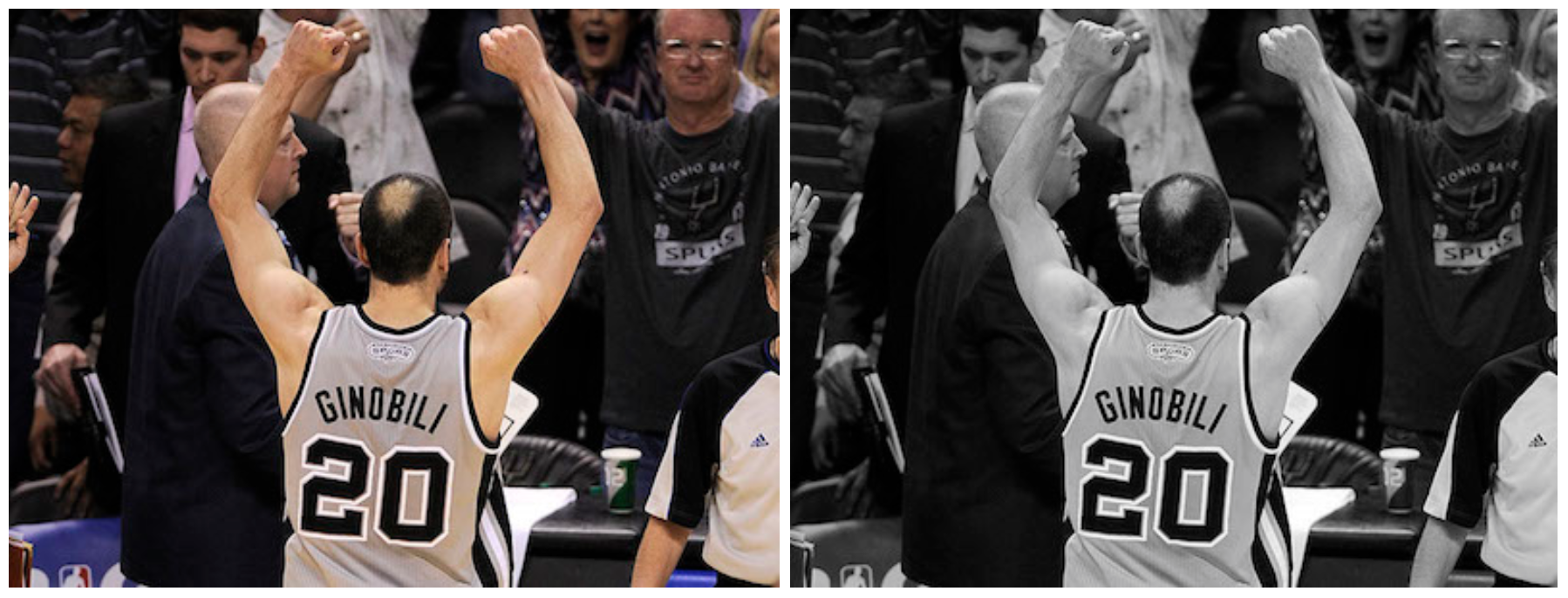Rather than coding from scratch, let us apply skimage.segmentation.felzenszwalb to the image.

import skimage.segmentation
from matplotlib import pyplot as plt

fig = plt.figure(figsize=(12, 5))
fig.suptitle("Felsenszwalb's efficient graph based image segmentation")
plt.tight_layout()
plt.show()


The code ran two versions of Felzenszwalb’s algorithms as shown in Fig. 8. The left k=100 generates a finer-grained segmentation with small regions where Manu’s bald spot is identified. The right one k=1000 outputs a coarser-grained segmentation where regions tend to be larger.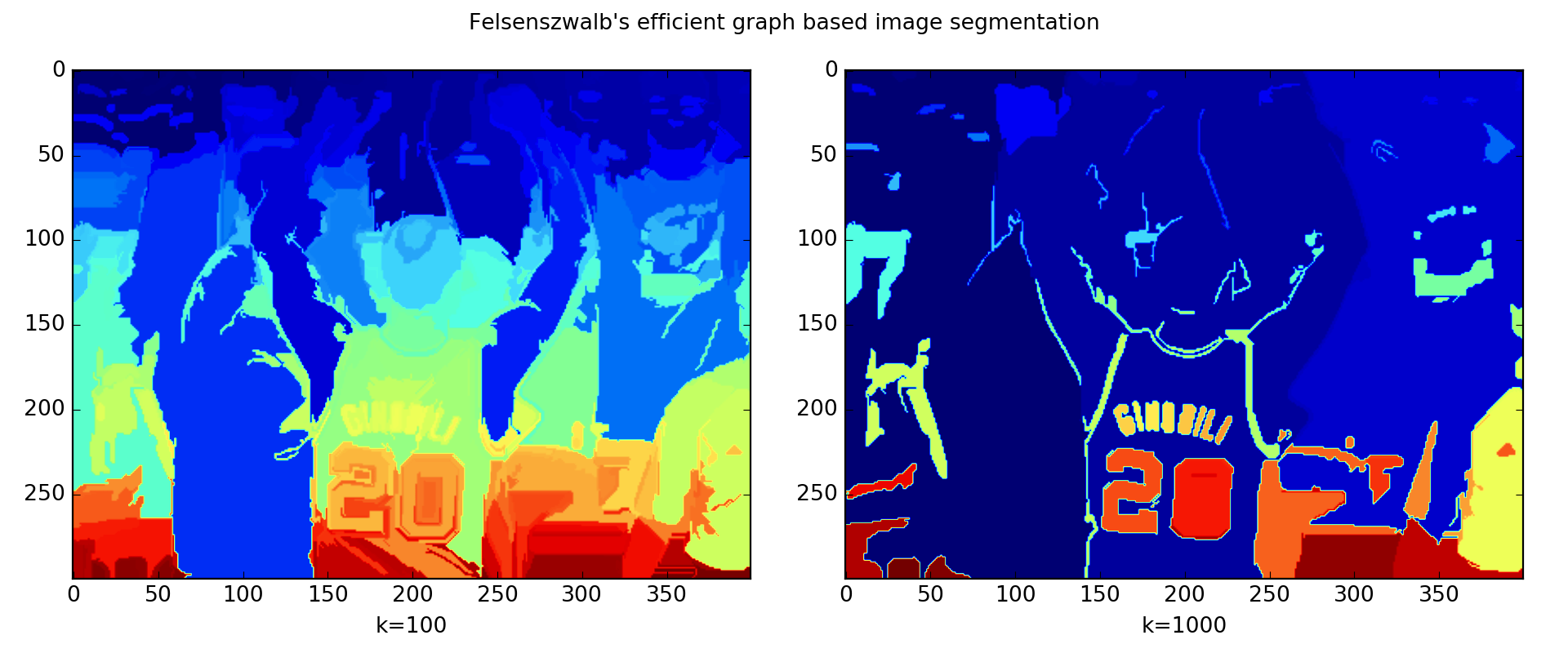# Selective Search#

Selective search is a common algorithm to provide region proposals that potentially contain objects. It is built on top of the image segmentation output and use region-based characteristics (NOTE: not just attributes of a single pixel) to do a bottom-up hierarchical grouping.

## How Selective Search Works#

1. At the initialization stage, apply Felzenszwalb and Huttenlocher’s graph-based image segmentation algorithm to create regions to start with.
2. Use a greedy algorithm to iteratively group regions together:
• First the similarities between all neighbouring regions are calculated.
• The two most similar regions are grouped together, and new similarities are calculated between the resulting region and its neighbours.
3. The process of grouping the most similar regions (Step 2) is repeated until the whole image becomes a single region.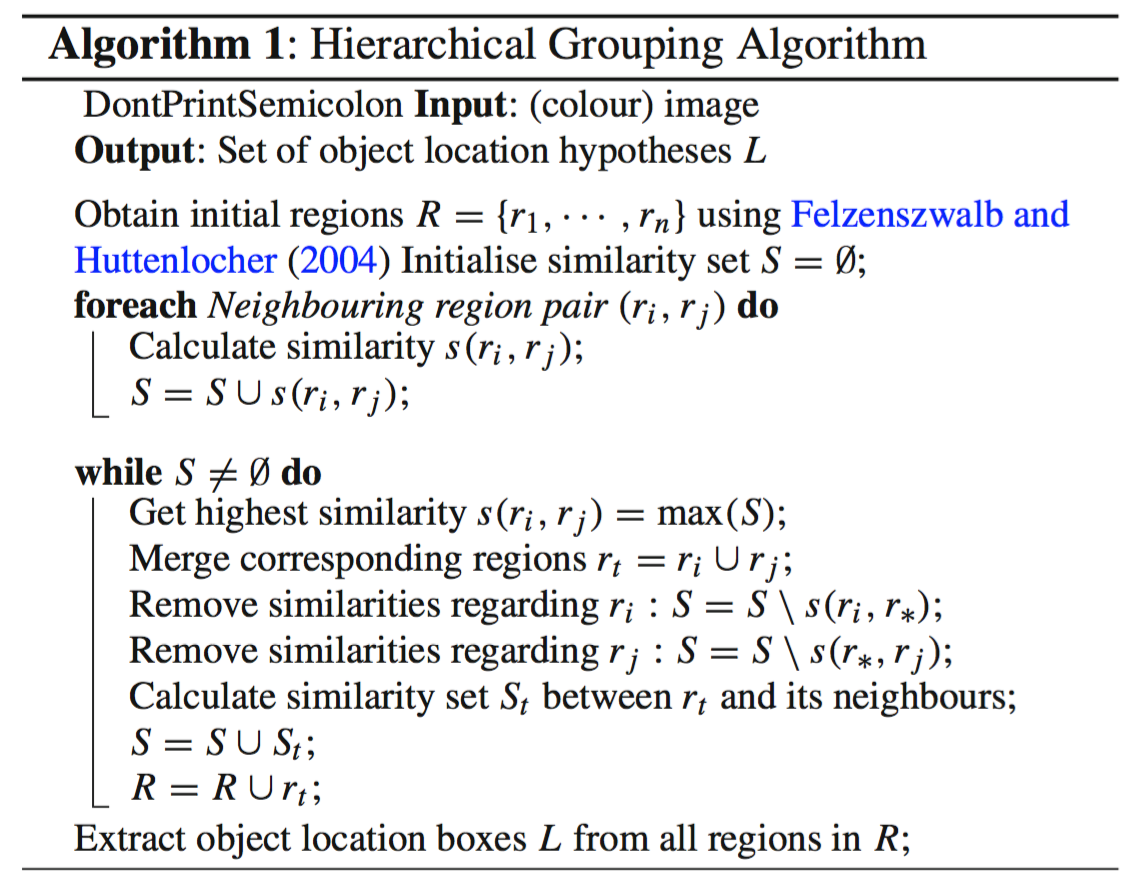## Configuration Variations#

Given two regions $(r_i, r_j)$, selective search proposed four complementary similarity measures:

• Color similarity
• Texture: Use algorithm that works well for material recognition such as SIFT.
• Size: Small regions are encouraged to merge early.
• Shape: Ideally one region can fill the gap of the other.

By (i) tuning the threshold $k$ in Felzenszwalb and Huttenlocher’s algorithm, (ii) changing the color space and (iii) picking different combinations of similarity metrics, we can produce a diverse set of Selective Search strategies. The version that produces the region proposals with best quality is configured with (i) a mixture of various initial segmentation proposals, (ii) a blend of multiple color spaces and (iii) a combination of all similarity measures. Unsurprisingly we need to balance between the quality (the model complexity) and the speed.

Cited as:

@article{weng2017detection1,
title   = "Object Detection for Dummies Part 1: Gradient Vector, HOG, and SS",
author  = "Weng, Lilian",
journal = "lilianweng.github.io",
year    = "2017",
url     = "https://lilianweng.github.io/posts/2017-10-29-object-recognition-part-1/"
}


 Dalal, Navneet, and Bill Triggs. “Histograms of oriented gradients for human detection.” Computer Vision and Pattern Recognition (CVPR), 2005.

 Pedro F. Felzenszwalb, and Daniel P. Huttenlocher. “Efficient graph-based image segmentation.” Intl. journal of computer vision 59.2 (2004): 167-181.